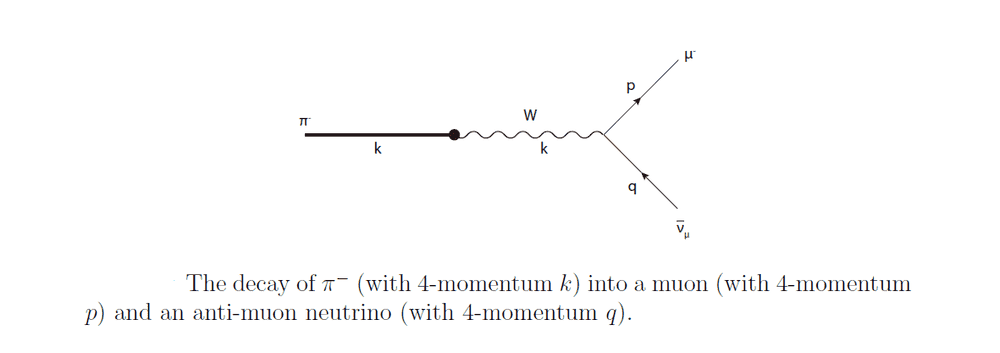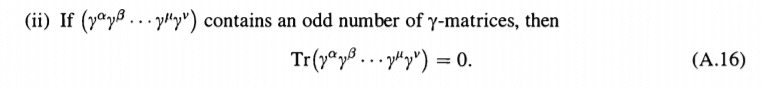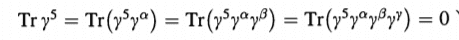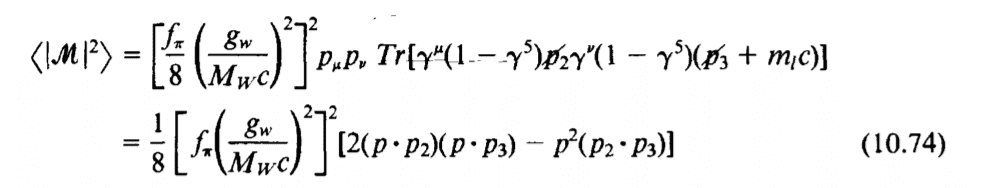# Pion Decay Rate

• I
Summary:
I am computing the decay rate for the pion into a muon and anti-muon neutrino but I do not get the desired expression *, so I would like to discuss the calculation
I am studying the following process: pion decays (mediated by the charged ##W## boson) into a muon and anti-muon neutrino (i.e. ##\pi^- \to \mu + \bar{\nu}_{\mu}##). The Feynman diagram associated to it isAnd its Feynman amplitude is (where we of course neglected ##\mathcal{O}(m_{\mu}^2/m_{W}^2)## and higher orders and assumed that the anti-muon neutrino is massless).

\begin{equation*}
\mathscr{M} = \frac{g^2}{8 m^2_W}f_{\pi} m_{\mu} \bar{u}_r(\vec p) (1-\gamma_5)v_s (\vec q)
\end{equation*}

I am particularly interested in verifying that the decay rate ##\Gamma## of such process is given by

\begin{equation*}
\Gamma = \frac{g^4 f_{\pi}^2}{256 \pi} \frac{m^2_{\mu}m_{\pi}}{m^4_{W}} \left( 1 - \frac{m^2_{\mu}}{m_{\pi}^2} \right)^2 \tag{*}
\end{equation*}

Let's get started. The differential decay rate is defined by ##d\Gamma := \left| S_{fi}\right|^2 / T##, where the matrix element ##S_{fi}## is given by

\begin{equation*}
S_{fi} = (2 \pi)^4 \delta^{(4)} (k - p - q) \sqrt{\frac{m}{V E_p}} \sqrt{\frac{m}{V E_q}} \sqrt{\frac{1}{2V E_q}} \sum_{r,s} \mathscr{M}
\end{equation*}

Let us first deal with the square of ##\mathscr{M}##; we have

\begin{align*}
\sum_{r,s} \left|\mathscr{M} \right|^2 &= \sum_{r,s} \frac{g^4}{64 m^4_W} f^2_{\pi} m_{\mu}^2 \underbrace{\bar u_r (\vec p) (1-\gamma_5) v_s(\vec q) \bar v_s(\vec q) (1-\gamma_5) u_r (\vec p)}_{\text{we can take the trace}} \\
&= \sum_{r,s} \frac{g^4}{64 m^4_W} f^2_{\pi} m_{\mu}^2 tr \left[\bar u_r (\vec p) (1-\gamma_5) v_s(\vec q) \bar v_s(\vec q) (1-\gamma_5) u_r (\vec p) \right] \\
&= \frac{g^4}{64 m^4_W} f^2_{\pi} m_{\mu}^2 tr \left[(1-\gamma_5) \underbrace{\sum_s v_s(\vec q) \bar v_s(\vec q)}_{(q \!\!/ - m_{\mu})/2m_{\mu}} (1-\gamma_5) \underbrace{\sum_r u_r (\vec p)\bar u_r (\vec p)}_{(q \!\!/ + m_{\mu})/2m_{\mu}} \right] \\
&= \frac{g^4}{256 m^4_W} f^2_{\pi} tr \left[(1-\gamma_5) (q \!\!/ - m_{\mu})(1-\gamma_5)(q \!\!/ + m_{\mu}) \right]
\end{align*}

My issue at this point is how to evaluate such trace.

Using the fact that ##\{ \gamma^{\mu}, \gamma^5 \} = 0## and the following propertiesI get

\begin{align*}
&tr\left[(1-\gamma_5)(q \!\!\!/ - m_{\mu})(1-\gamma_5)(p \!\!\!/ + m_{\mu}) \right]\\
&= tr\left[ (q \!\!\!/ (1 + \gamma_5) - m_{\mu}(1 - \gamma_5)) (1-\gamma_5)(p \!\!\!/ + m_{\mu}) \right] \\
&= tr\left[ - m_{\mu}(1 - \gamma_5)^2 (p \!\!\!/ + m_{\mu}) \right] \\
&= -2tr\left[ m_{\mu}(1 - \gamma_5) (p \!\!\!/ + m_{\mu})\right] \\
&= -8 m_{\mu}^2
\end{align*}

However, this does not lead to (*). It seems suspicious to me that momenta terms vanish...

Do you see any mistake so far? If not, the mistake has to be in what follows so I will post the rest of the calculation.

Thank youPS: I post this specific calculation here because it is directly related to particle physics and I had really good previous experiences in this forum thanks to vanhees71, nrqed & Dr.AbeNikIanEdL

Staff Emeritus
Chapter 10 of Griffiths goes through this. FWIW, I don't follow your logic in your last five rows of TeX. Terms seem to vanish before you have demonstrated that they have an odd number of gamma matrices. Maybe they are zero, but I can't see it from what you have written. You might want to expand to all 16 terms before you start cancelling.

•JD_PM

To get to the first equality I used ##\{ \gamma^{\mu}, \gamma^5 \} = 0##, so that we get
##(1-\gamma_5)(q \!\!\!/ - m_{\mu}) = q \!\!\!/ + \gamma_5 m_{\mu} - m_{\mu} -\gamma_5 q \!\!\!/ = q \!\!\!/ + \gamma_5 m_{\mu} - m_{\mu} + q \!\!\!/ \gamma_5 =
q \!\!\!/ (1 + \gamma_5) - m_{\mu}(1 - \gamma_5)##

To get to the second equality I used the fact that ##\gamma_5^2 = 1## so that one of the terms cancels due to
##(1 + \gamma_5)(1 - \gamma_5) = 1 - \gamma_5^2 = 0##

To get to the third equality I used ##(1- \gamma_5)^2 = 2(1- \gamma_5)##

Finally, traces with odd number of gamma matrices vanish. Traces containing ##\gamma^5## or ##\gamma^5 \gamma^{\mu}## vanish as well. Hence, only ##-2m_{\mu}^2 tr(\Bbb 1_{4 \times 4} ) = -8m_{\mu}^2 ## survives.

Staff Emeritus
I am 99% sure you have dropped a sign somewhere. You want a p-slash times q-slash in the answer, right? But that piece gets zeroed out.

Like I said, Griffiths works this all out. It will be a lot more efficient and instructive for you to follow him than to have us searching for minus signs.

Like I said, Griffiths works this all out.

Thanks for the reference! I will study it and if I still have questions I will come back :)

nrqed
Homework Helper
Gold Member
Summary:: I am computing the decay rate for the pion into a muon and anti-muon neutrino but I do not get the desired expression *, so I would like to discuss the calculation

I am studying the following process: pion decays (mediated by the charged ##W## boson) into a muon and anti-muon neutrino (i.e. ##\pi^- \to \mu + \bar{\nu}_{\mu}##). The Feynman diagram associated to it is

View attachment 279894

And its Feynman amplitude is (where we of course neglected ##\mathcal{O}(m_{\mu}^2/m_{W}^2)## and higher orders and assumed that the anti-muon neutrino is massless).

\begin{equation*}
\mathscr{M} = \frac{g^2}{8 m^2_W}f_{\pi} m_{\mu} \bar{u}_r(\vec p) (1-\gamma_5)v_s (\vec q)
\end{equation*}
You forgot a ##\gamma_\mu##!

•JD_PM and vanhees71
You forgot a ##\gamma_\mu##!

Oops, indeed!By the way, the trace I wrote down in the OP is not the one we are looking for. I have corrected my original mistake but I would still like to check a detail of my computation

\begin{align*}
&p'_{\mu}p'_{\nu} tr\left[\gamma^{\mu}(1-\gamma_5)p \!\!\!/ \gamma^{\nu}(1-\gamma_5)(q \!\!\!/ + m_{\mu}) \right]\\
&= 8 p'_{\mu}p'_{\nu} \left[ p^{\mu}q^{\nu} + p^{\nu}q^{\mu} - \eta^{\mu \nu} \left( p \cdot q\right) - i \varepsilon^{\mu \nu \rho \sigma}p_{\rho} q_{\sigma} \right] \\
&= 8 \left[ 2(p' \cdot p)(p' \cdot q) - p'^2\left( p \cdot q\right) -ip'_{\mu}p'_{\nu} \varepsilon^{\mu \nu \rho \sigma}p_{\rho} q_{\sigma}\right] \\
&= 8 \left[ 2(p' \cdot p)(p' \cdot q) - p'^2\left( p \cdot q\right) -ip'_{\mu}p'_{\lambda} \varepsilon^{\mu \lambda \lambda \sigma}p_{\lambda} q_{\sigma}\right] \\
&= 8 \left[ 2(p' \cdot p)(p' \cdot q) - p'^2\left( p \cdot q\right) \right] \\
\end{align*}

So is it OK to justify that the ##\varepsilon## vanishes due to relabeling two of the dummy indices as above ( given that repeating two of the indices of ##\varepsilon## makes it vanish by definition)? Or is there another reason?

vanhees71
Gold Member
You cannot have one index more then twice in a formula using the Ricci calculus!

•JD_PM
You cannot have one index more then twice in a formula using the Ricci calculus!

Thanks for clarifying vanhees71

Then I do not see why ##ip'_{\mu}p'_{\nu} \varepsilon^{\mu \nu \rho \sigma}p_{\rho} q_{\sigma}## term vanishes...By the way, my trace computation matches Griffiths'vanhees71
Gold Member
It vanishes, because ##\epsilon^{\mu \nu \rho \sigma} p_{\mu}' p_{\nu}'=0##, contracting the antisymmetric Levi-Civita symbol with an expression symmetric in the indices ##\mu## and ##\nu##.

•JD_PM
Gosh, that was a big miss of mine! Thanks again vanhees71

Staff Emeritus
Gosh, that was a big miss of mine!

Recommendation:
1. Expand it all out. Paper is cheap. Electrons even cheaper.
2. Then remove terms that are zero automatically (e.g. odd number of gamma matrices)
3. Then remove terms that cancel other terms.
4. Consolidate. Put all the epsilon tensors together.
5. Look for ways to cancel what's left - often a set of epsilon tensors sum to zero, for example.
6. Then look for simple expressions, e. g. $p_1 \cdot p_2$
Keep an eye out for physical intuition: does your rate depend on whether your interaction is V+A or V-A? If not, your calculation shouldn't either.

•JD_PM